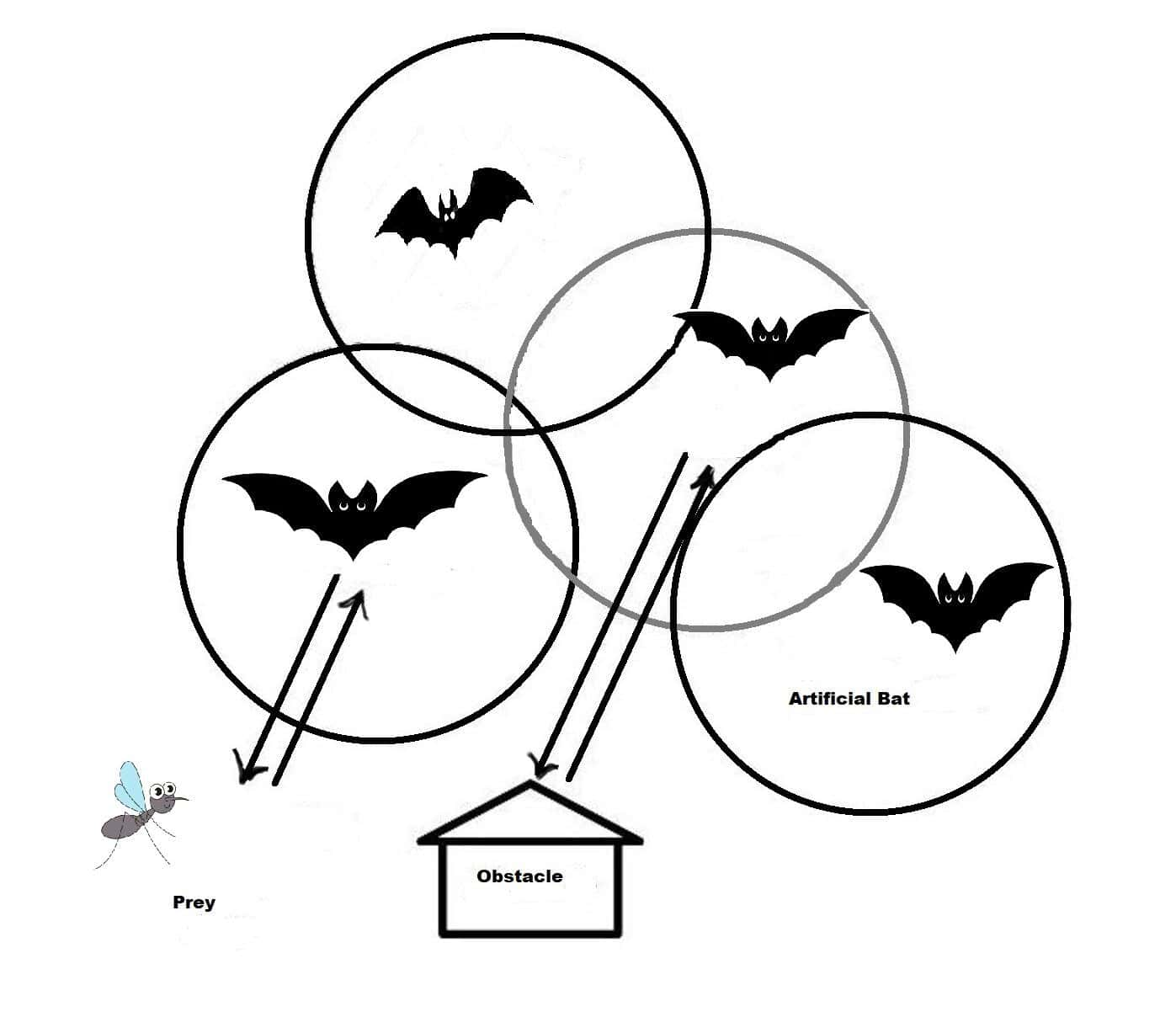## 1. Overview

The Bat algorithm is a population-based metaheuristics algorithm for solving continuous optimization problems. It’s been used to optimize solutions in cloud computing, feature selection, image processing, and control engineering problems.

In this tutorial, we’ll learn about meta-heuristics and the Bat algorithm. But, before doing this, let’s take a quick look at meta-heuristics.

## 2. Metaheuristics

Metaheuristics are similar to heuristics, and they seek promising solutions to problems. However, metaheuristics are generic and can deal with a variety of problems.

In the context of metaheuristics, the characteristics of “non-measurable success” and “reasonable execution” remain the same as discussed for heuristics. But metaheuristics replace the problem-based design principle with the problem-independent design principle. Generally, metaheuristic algorithms are designed for global optimization.

## 3. Bat Algorithm

Yang proposed the Bat algorithm in 2010. The basic Bat algorithm is bio-inspired. It’s based on the characteristics of bat bio-sonar or echolocation. Bats in the wild emit ultrasonic waves into the environment to aid in hunting or navigation.

Following the emission of these waves, it receives the echoes of the waves. It locates itself and identifies obstacles by using the received echoes.

It’s illustrated in the following figure:Furthermore, each agent in the swarm can locate the most “nutritious” areas or return to the swarm’s previous best location. The Bat algorithm has demonstrated excellent efficiency in solving continuous optimization problems.

## 4. Steps of the Bat Algorithm

The flowchart of the Bat algorithm is given as follows:We must first initialize the bat population before defining the pulse frequency. After this, we define the maximum number of iterations and initialize pulse rates and loudness.

If the result is better, new values will be generated, and values for velocities will be updated. If the solution is yes, then we must choose the best solution from these random values. If not, the program will return.

When new solutions appear as random values, it accepts them and searches for the best current value to output.

## 5. Coding for Bat Algorithm

After understanding the concept and steps of the Bat algorithm, let’s look at the pseudocode of the Bat algorithm:We’ve multiple implementations of the Bat algorithm in different programming languages, such as Python and Matlab.

## 6. Use Cases of the Bat Algorithm

Feature selection is a data pre-processing method used in classification. The main goal is to minimize the number of characteristics while improving classification performance. The binary vector solves the problem of which features to select or not select in a specific problem. In this case, 0 indicates no selection, and 1 indicates that the feature for the provided data should be chosen.

In digital image processing, thresholding is the easiest way to segment images. The Bat algorithm is used to perform multilevel image thresholding in this context.

Bat algorithm is applied on brushless DC wheel motor problem in order to optimize the parameters.

Load frequency control using Bat algorithm is applied in the relevant literature. It is used to schedule PI controllers for interconnected power systems.

Bat algorithm is applied in order to optimize fuel in the reactor core.

## 7. Complexity of Bat Algorithm

Generally, the time complexity of meta-heuristic algorithms that search for global optimization can’t be found. Since these algorithms don’t guarantee to find the global optima solution within a given time limit, it’s impossible to find time complexity.

On the other hand, the best way to compare the complexity of metaheuristic algorithms is to know time and space requirements by using computational complexity theory. We must keep in mind that this is very difficult for stochastic algorithms. Computational complexity of an algorithm refers to the amount of resources required to run the relevant algorithm.

Computational complexity of the Bat algorithm is. In this equation,is the number of operations on bits that are needed to run the algorithm.is the size of the input.refers to time. It is not affected by the size of the input on a relevant machine and is often called as steps.

## 8. Conclusion

In this tutorial, we learned about a meta-heuristic algorithm called the Bat algorithm. First and foremost, we refreshed our knowledge about meta-heuristics. Then, we looked into the implementation steps of the Bat algorithm. Finally, we discussed the use cases of the Bat algorithm.